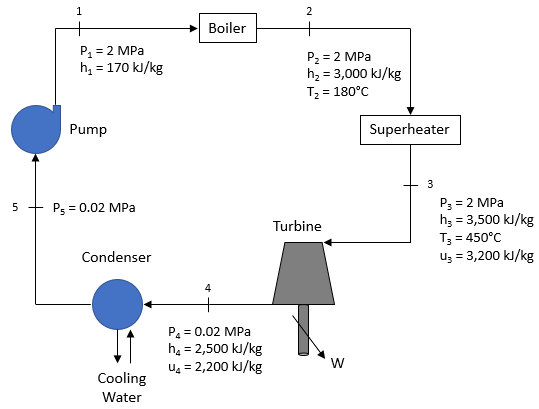## Turbine Power

The Rankine cycle below is used to power a plant complex with water as the working fluid. If the fluid’s mass flow rate is 25 kg/s, what is the turbine’s output power in MW? Assume steady state and steady flow conditions. Also, disregard pressure losses and kinetic/potential energy effects. Note the density of water is 1,000 kg/m^3.Hint
Turbines, Pumps, and Compressors are often considered adiabatic (no heat transfer), so in a steady-state and steady-flow conditions:
$$h_{in}=h_{exit}+w$$$where $$h$$ is specific enthalpy, and $$w$$ is the turbine work per unit mass. Hint 2 $$\dot{W}=w\times \dot{m}$$$
where $$\dot{W}$$ is the power, $$w$$ is the work per unit mass, and $$\dot{m}$$ is the mass flow rate.
Turbines, Pumps, and Compressors are often considered adiabatic (no heat transfer), so in a steady-state and steady-flow conditions:
$$h_{in}=h_{exit}+w$$$where $$h$$ is specific enthalpy, and $$w$$ is the work per unit mass. Per the problem statement’s figure: $$w_{turbine}=h_3-h_4=3,500-2,500=1,000\:kJ/kg$$$
Thus, to get the power produced by the turbine:
$$\dot{W}=w_{turbine}\times \dot{m}=1,000\frac{kJ}{kg}\times 25\frac{kg}{s}=25,000\:kW=25\:MW$$\$
25 MW